# Alcohol

How many cubic centimeters of 96% alcohol is needed to obtain 0.75 liters of 40% alcohol?

x =  312.5

### Step-by-step explanation:

0.96 x = (0.75•1000) • 0.40

0.96•x = (0.75•1000) • 0.40

0.96x = 300

x = 625/2 = 312.5

Our simple equation calculator calculates it.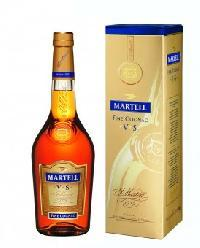Did you find an error or inaccuracy? Feel free to write us. Thank you!Tips to related online calculators
Do you have a linear equation or system of equations and looking for its solution? Or do you have a quadratic equation?
Do you know the volume and unit volume, and want to convert volume units?

## Related math problems and questions:

• AlcoholHow many 55% alcohol we need to pour into 14 liters 75% alcohol to get p3% alcohol? How many 65% alcohol we get?
• Alcohol mixtureThree liters of 96 percent alcohol set up a certain amount of distilled water to form 54-percent alcohol. How many liters of distilled water was used?
• Alcohol mixing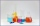How much 64% of the alcohol must be poured into 6 liters of 85% alcohol to produce 79% alcohol?
• Pouring alcohol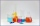100 liters of alcohol has 70% How many liters of water needs to be added to have 60% alcohol?
• Alcohol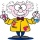6l 48% alcohol, how much 52% alcohol must be added to give 50% alcohol?
• Alcohol solutionsWe have to produce 2 liters of 60% alcohol from 55% and 80%. How many of which ones will we use in the solution?
• Solutions, mixturesHow many liters of 70% solution must we add to 5 liters of 30% solution to give us a 60% solution?
• PharmacyThe pharmacy adds to 3 liters of 95-percent alcohol 5 liters of 38.04 percent alcohol. How much percent alcohol the pharmacy got?
• SolutionsHow much 60% solution and how much 35% solution is needed to create 100 l of 40% solution?
• Solutions, mixturesWe have 2 liters of 20% solution available. How much 70% solution do we need to add to it to get a 30% solution?
• Alcohol mixtureFrom 55% and 80% alcohol, we have to produce 0.2 kg of 60% alcohol. How many of them do we use in the solution?
• Alcohol 2Two types of alcohol one 63% and second 75% give 20 liters of 69% alcohol. How many liters of each type are in the mixture?
• Lions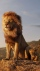If 8 lions is 40% how many would 75% be?
• Mixture 2How many liters of water must be added to 7 liters of a 20% solution to obtain a 10% solution?
• Acid solutionBy adding 250 grams of a 96% sulfuric acid solution to its 3% solution, we changed its initial concentration to 25%. How many grams of 3% of the acid was used for dilution?
• SolutionWhat amount of 80% solution and what amount of water is needed to mix in order to obtain two liters of 60% solution of this mixture?
• Concentration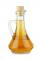How 0.5 liters of 8% vinegar diluted to a concentration of 20 hundredths % of vinegar? How many liters of water must be poured?